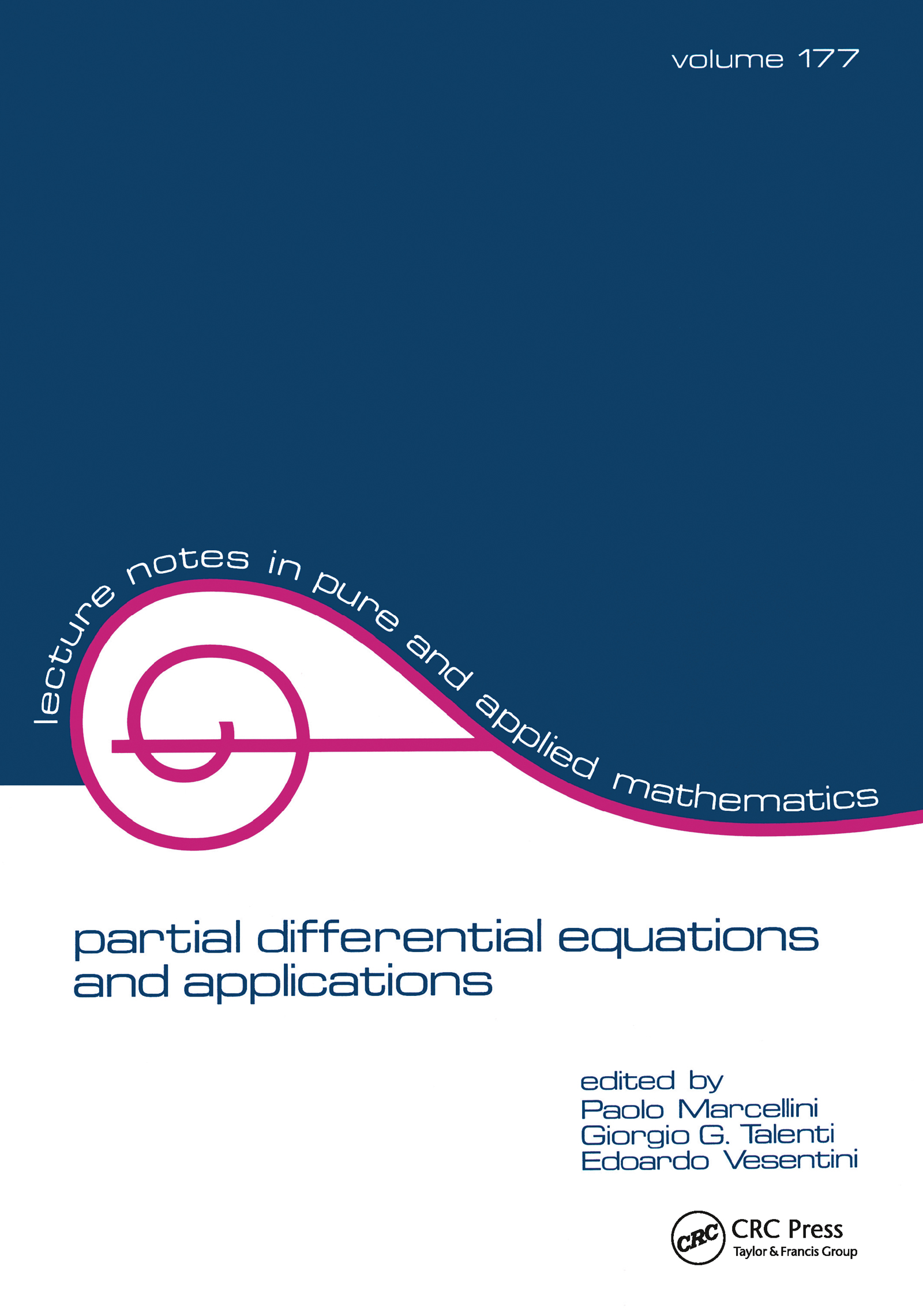# partial differential equations and applications

## Collected Papers in Honor of Carlo Pucci, 1st Edition

CRC Press

392 pages

##### Purchasing Options:\$ = USD
Paperback: 9780824796983
pub: 1996-01-16
SAVE ~\$60.00
\$300.00
\$240.00
x
Hardback: 9781138417571
pub: 2017-08-15
SAVE ~\$41.00
\$205.00
\$164.00
x
eBook (VitalSource) : 9780203744369
pub: 2017-10-02
from \$150.00

FREE Standard Shipping!

### Description

Written as a tribute to the mathematician Carlo Pucci on the occasion of his 70th birthday, this is a collection of authoritative contributions from over 45 internationally acclaimed experts in the field of partial differential equations. Papers discuss a variety of topics such as problems where a partial differential equation is coupled with unfavourable boundary or initial conditions, and boundary value problems for partial differential equations of elliptic type.

### Table of Contents

Spatial decay estimates for an evolution equation; on the range of Ural'tseva's axially symmetric operator in Sobolev spaces; the use of a priori information in the solution of ill-posed problems; allocation maps with general cost functions; an elementary theorem in plane geometry and its multidimensional extension; minimum problems for volumes of convex bodies; on the continuous dependence of the solution of a linear parabolic partial differential equation on the boundary data and the solution at an interior spatial point; decomposability of rectangular and triangular probability distributions; nonlinear infinite networks with nonsymmetric resistances; about a singular parabolic equation arising in thin film dynamics and in the Ricci flow for complete IR2; alternating-direction iteration for the p-version of the finite element method; an integrodifferential analog of semilinear parabolic PDE's; on solutions of mean curvature type inequalities; an application of the calculus of variations to the study of optimal foraging; a limit model of a soft thin joint; projective invariants of complete intersections; m-hyperbolicity, evenness and normality; an extended variational principle; instability criteria for solutions of second order elliptic quasilinear differential equations; maximum principles for difference operators; a generic uniqueness result for the Stokes system and its control theoretical consequences; on a Stefan problem in a concentrated capacity; total total internal reflection; the reflector problem for closed surfaces; upper bounds for Eigenvalues of elliptic operators; stability for abstract evolution equations; new techniques in critical point theory; detecting underground gas sources; conservative operators; on the regularization of the antenna synthesis problem; the problem of packaging; the first digit problem and scale-invariance; change of variable in the SL-integral; the minimum energy configuration of a mixed-material column.

### Subject Categories

##### BISAC Subject Codes/Headings:
MAT007000
MATHEMATICS / Differential Equations